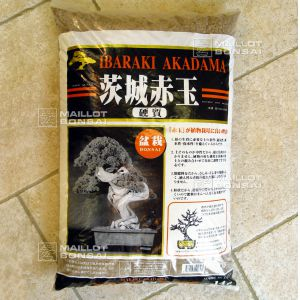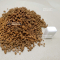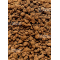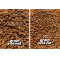##### The Japanese Bonsai specialist
Direct order Contact Help / Services Newsletter# Akadama multi-purpose bonsai soil 14ltr bag

› Bonsai soil and fertiliser › Bonsai soilref. : 6374

26,00

voluminous/heavy item extra shipping of 5,00

Available quantity : 41Order

###### Description

Multi-purpose medium grain bonsai soil used to grow many species.

Grain size Ø -+1/3/ 1/5 mm. One bag +- 14 litre. SUPERIOR QUALITY, DRIED FOR MORE THAN 2 YEARS. Weight according to the rate of moisture of 8.15 kilograms.

Origin: natural volcanic earth from the mountainous regions of Japan.

Benefits: soil drainage and root ventilation for the healthy growth of your bonsai.

How to use:use alone or add gravel and large-grained sand but not compost. This earth is not very nourishing and a Biogold fertiliser should be used regularly. You'll need to water this large-grained soil more in summer.

Species: for all deciduous trees, conifer and caduc trees (for rhododendrons and azaleas you'll need Kanuma earth).

Please kindly note that this is a heavy product to send and we charge extra for postage.

#soil 7.4 #bonsai 6.6 #purpose 3.7 #earth 3.5 #multi 3.5 #this 3.4 #species 2.7 #grained 2.7 #akadama 2.7 #trees 2.5

Formule
(( ROUND((CHAR_LENGTH(b.article_nom)-CHAR_LENGTH(REPLACE(b.article_nom, 'soil', '')))/LENGTH('soil')) + ROUND((CHAR_LENGTH(b.article_description)-CHAR_LENGTH(REPLACE(b.article_description, 'soil', '')))/LENGTH('soil')) ) * 5.4) + (( ROUND((CHAR_LENGTH(b.article_nom)-CHAR_LENGTH(REPLACE(b.article_nom, 'bonsai', '')))/LENGTH('bonsai')) + ROUND((CHAR_LENGTH(b.article_description)-CHAR_LENGTH(REPLACE(b.article_description, 'bonsai', '')))/LENGTH('bonsai')) ) * 4.6) + (( ROUND((CHAR_LENGTH(b.article_nom)-CHAR_LENGTH(REPLACE(b.article_nom, 'purpose', '')))/LENGTH('purpose')) + ROUND((CHAR_LENGTH(b.article_description)-CHAR_LENGTH(REPLACE(b.article_description, 'purpose', '')))/LENGTH('purpose')) ) * 3.7) + (( ROUND((CHAR_LENGTH(b.article_nom)-CHAR_LENGTH(REPLACE(b.article_nom, 'earth', '')))/LENGTH('earth')) + ROUND((CHAR_LENGTH(b.article_description)-CHAR_LENGTH(REPLACE(b.article_description, 'earth', '')))/LENGTH('earth')) ) * 3.5) + (( ROUND((CHAR_LENGTH(b.article_nom)-CHAR_LENGTH(REPLACE(b.article_nom, 'multi', '')))/LENGTH('multi')) + ROUND((CHAR_LENGTH(b.article_description)-CHAR_LENGTH(REPLACE(b.article_description, 'multi', '')))/LENGTH('multi')) ) * 3.5) + (( ROUND((CHAR_LENGTH(b.article_nom)-CHAR_LENGTH(REPLACE(b.article_nom, 'this', '')))/LENGTH('this')) + ROUND((CHAR_LENGTH(b.article_description)-CHAR_LENGTH(REPLACE(b.article_description, 'this', '')))/LENGTH('this')) ) * 3.4) + (( ROUND((CHAR_LENGTH(b.article_nom)-CHAR_LENGTH(REPLACE(b.article_nom, 'species', '')))/LENGTH('species')) + ROUND((CHAR_LENGTH(b.article_description)-CHAR_LENGTH(REPLACE(b.article_description, 'species', '')))/LENGTH('species')) ) * 2.7) + (( ROUND((CHAR_LENGTH(b.article_nom)-CHAR_LENGTH(REPLACE(b.article_nom, 'grained', '')))/LENGTH('grained')) + ROUND((CHAR_LENGTH(b.article_description)-CHAR_LENGTH(REPLACE(b.article_description, 'grained', '')))/LENGTH('grained')) ) * 2.7) + (( ROUND((CHAR_LENGTH(b.article_nom)-CHAR_LENGTH(REPLACE(b.article_nom, 'akadama', '')))/LENGTH('akadama')) + ROUND((CHAR_LENGTH(b.article_description)-CHAR_LENGTH(REPLACE(b.article_description, 'akadama', '')))/LENGTH('akadama')) ) * 2.7) + (( ROUND((CHAR_LENGTH(b.article_nom)-CHAR_LENGTH(REPLACE(b.article_nom, 'trees', '')))/LENGTH('trees')) + ROUND((CHAR_LENGTH(b.article_description)-CHAR_LENGTH(REPLACE(b.article_description, 'trees', '')))/LENGTH('trees')) ) * 2.5)

## Secure payment## Delivery

Our logistic partners :04 74 55 23 48
Pépinière MAILLOT-BONSAÏ
Le Bois Frazy
01990 RELEVANT - FRANCE
on appointment